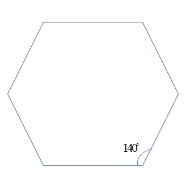One angle of a six sided polygon is ${{140}^{\circ }}$ and the other angles are equal. Find the measure of each angle.(a) ${{116}^{\circ }}$ (b) ${{126}^{\circ }}$ (c) ${{112}^{\circ }}$ (d) ${{118}^{\circ }}$Verified
147.3k+ views
Hint: First, firstly we will draw the six sided polygon which is called a hexagon with one of its angle as ${{140}^{\circ }}$ and all other angles are equal. Then, we know the formula for the sum of the interior angles of a polygon where n is the number of sides is given by $180\left( n-2 \right)$ . Then, by substituting the value of n as 6 and by using the above condition, we get the sum of all the angles of the given polygon, we get the desired answer.

In this question, we are supposed to find the measure of each angle when one angle of a six sided polygon is ${{140}^{\circ }}$.
Now, firstly we will draw the six sided polygon which is called a hexagon with one of its angles as ${{140}^{\circ }}$ and all other angles are equal.So, the condition is given that all the remaining angles are equal and let us consider its angle as x.
Now, we know the formula for the sum of the interior angles of a polygon where n is the number of sides is given by:
$180\left( n-2 \right)$
Then, here we have the polygon with 6 sides so the value of n is 6.
Then, we will substitute the value of n in above formula as:
\begin{align} & 180\left( 6-2 \right)=180\times 4 \\ & \Rightarrow {{720}^{\circ }} \\ \end{align}
So, the sum of all the interior angles of a six sided polygon is ${{720}^{\circ }}$ .
Now, by using the above condition, we get the sum of all the angles of the given polygon is:
${{140}^{\circ }}+5\times x={{720}^{\circ }}$
Now, solve the above expression to get the value of x which is the measure of angle of the polygon as:
\begin{align} & 5\times x={{720}^{\circ }}-{{140}^{\circ }} \\ & \Rightarrow 5x={{580}^{\circ }} \\ & \Rightarrow x=\dfrac{{{580}^{\circ }}}{5} \\ & \Rightarrow x={{116}^{\circ }} \\ \end{align}
So, the remaining angles of the polygon which are equal have the angle equal to ${{116}^{\circ }}$ .
So, the correct answer is “Option A”.

Note: Now, to solve these types of problems we must be careful with the calculations as there is not much chance of error unless we don’t know the formula for the sum of interior angles of the n sided polygon is given by $180\left( n-2 \right)$ . So ,this is to be kept in mind before solving these kinds of problems.